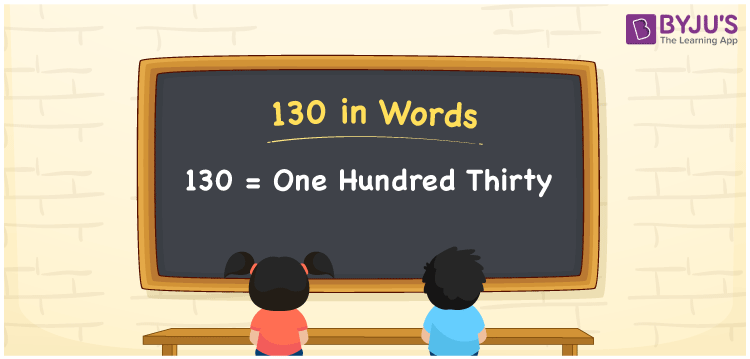# 130 in Words

130 in words is written as One Hundred Thirty. The name of number 130 in English is “One Hundred Thirty”. The word One Hundred Thirty is used in many real-life situations. For example, there are One Hundred Thirty people in a church. Thus, it is a counting number. Also, to represent the currency equal to 130, we can write it in words as Rupees One Hundred Thirty or One Hundred Thirty rupees. Therefore, it is necessary to learn the numbers in words, for the ease of understanding and expressing them.

 130 in words One Hundred Thirty One Hundred Thirty in Numerals 130

## 130 in English Words## How to Write 130 in Words?

If we know the place values of the number 130, then we can easily express it in words. The place value is basically the position of a digit in a number. 130 is a three-digit number, therefore, we can specify the position of each digit of 130 in a place value chart. In the Indian numbering system, the order of place value of digits from right to left is given by:

 Hundreds Tens Ones 1 3 0

We can write it in expanded form as:

1 × Hundred + 3 × Ten + 0 × One

= 1 × 100 + 3 × 10 + 0 × 1

= 100 + 30

= 130

= One Hundred Thirty

Hence, 130 in words is written as One Hundred Thirty.

130 is a natural number that precedes 131 and succeeds 129.

130 in words – One Hundred Thirty

Is 130 an odd number? – No

Is 130 an even number? – Yes

Is 130 a perfect square number? – No

Is 130 a perfect cube number? – No

Is 130 a prime number? – No

Is 130 a composite number? – Yes

## Frequently Asked Questions on 130 in Words

Q1

### How to write 130 in words?

130 in English is written as “One Hundred Thirty”.
Q2

### Is 130 an odd number or even number?

130 is an even number because it is wholly divisible by 2.
130/2 = 65
Q3

### Write One Hundred Thirty in numbers.

One Hundred Thirty in numbers is 130.
Test your Knowledge on 130 in Words